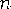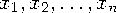Functions and CALL Routines

# HARMEANZ Function

Returns the harmonic mean, using zero fuzzing.
 Category: Descriptive Statistics

## Syntax

 HARMEANZ(argument<,argument,...>)

### Arguments

argument

is a non-negative numeric constant, variable, or expression.

 Tip: The argument list can consist of a variable list, which is preceded by OF.

The MEAN function returns the arithmetic mean (average), and the GEOMEAN function returns the geometric mean, whereas the HARMEANZ function returns the harmonic mean of the non-missing values. Unlike HARMEAN, HARMEANZ does not fuzz the values of the arguments that are approximately zero.

If any argument is negative, then the result is a missing value. A message appears in the log that the negative argument is invalid, and _ERROR_ is set to 1. If all the arguments are missing values, then the result is a missing value. Otherwise, the result is the harmonic mean of the non-missing values.

If any argument is zero, then the harmonic mean is zero. Otherwise, the harmonic mean is the reciprocal of the arithmetic mean of the reciprocals of the values.

Letbe the number of arguments with non-missing values, and letbe the values of those arguments. The harmonic mean isSAS Statements Results
`x1=harmeanz(1,2,4,4);`
`2`
`x2=harmeanz(.,4,12,24);`
`8`
`x3=harmeanz(of x1-x2);`
`3.2`

 Function:Previous Page | Next Page | Top of Page